# Visualizing the Capital Asset Pricing Model

by Jonathan Regenstein

In a previous post, we covered how to calculate CAPM beta for our usual portfolio consisting of:

+ SPY (S&P500 fund) weighted 25%
+ EFA (a non-US equities fund) weighted 25%
+ IJS (a small-cap value fund) weighted 20%
+ EEM (an emerging-mkts fund) weighted 20%
+ AGG (a bond fund) weighted 10%

Today, we will move on to visualizing the CAPM beta and explore some ggplot and highcharter functionality, along with the broom package.

Before we can do any of this CAPM work, we need to calculate the portfolio returns, covered in this post, and then calculate the CAPM beta for the portfolio and the individual assets covered in this post.

I will not present that code or logic again but we will utilize four data objects from that previous work:

+ portfolio_returns_tq_rebalanced_monthly (a tibble of portfolio monthly returns)
+ market_returns_tidy (a tibble of SP500 monthly returns)
+ beta_dplyr_byhand (a tibble of market betas for our 5 individual assets)
+ asset_returns_long (a tibble of returns for our 5 individual assets)

Let’s get to it.

### Visualizing the Relationship between Portfolio Returns, Risk and Market Returns

The CAPM beta number is telling us about the linear relationship between our portfolio returns and the market returns. It’s also telling us about the riskiness of our portfolio - how volatile the portfolio is relative to the market. Before we get to beta itself, let’s take a look at expected monthly returns of our assets scattered against monthly risk of our individual assets.

library(tidyquant)
library(tidyverse)
library(timetk)
library(tibbletime)
library(scales)

# This theme_update will center your ggplot titles
theme_update(plot.title = element_text(hjust = 0.5))

asset_returns_long %>%
group_by(asset) %>%
summarise(expected_return = mean(returns),
stand_dev = sd(returns)) %>%
ggplot(aes(x = stand_dev, y = expected_return, color = asset)) +
geom_point(size = 2) +
ylab("expected return") +
xlab("standard deviation") +
ggtitle("Expected Monthly Returns v. Risk") +
scale_y_continuous(label = function(x){ paste0(x, "%")})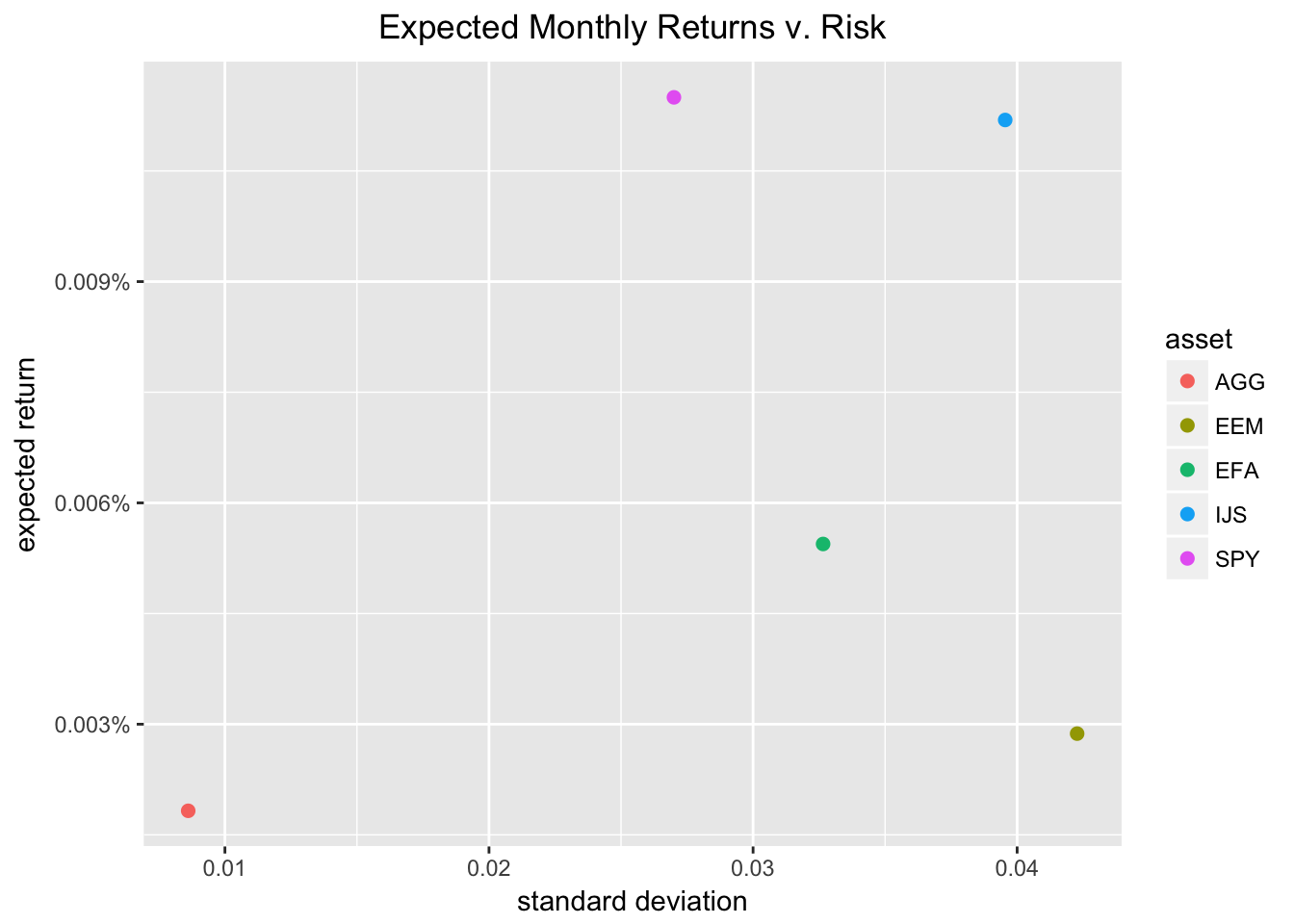Where does our portfolio fit on this scatter plot? Let’s add it to the ggplot() flow with geom_point(aes(x = sd(portfolio_returns_tq_rebalanced_monthly$returns), y = mean(portfolio_returns_tq_rebalanced_monthly$returns)), color = "cornflowerblue", size = 3).

asset_returns_long %>%
group_by(asset) %>%
summarise(expected_return = mean(returns),
stand_dev = sd(returns)) %>%
ggplot(aes(x = stand_dev, y = expected_return, color = asset)) +
geom_point(size = 2) +
geom_point(aes(x = sd(portfolio_returns_tq_rebalanced_monthly$returns), y = mean(portfolio_returns_tq_rebalanced_monthly$returns)),
color = "cornflowerblue",
size = 3) +
geom_text(
aes(x = sd(portfolio_returns_tq_rebalanced_monthly$returns) * 1.09, y = mean(portfolio_returns_tq_rebalanced_monthly$returns),
label = "portfolio")) +
ylab("expected return") +
xlab("standard deviation") +
ggtitle("Expected Monthly Returns v. Risk") +
scale_y_continuous(labels = function(x){ paste0(x, "%")})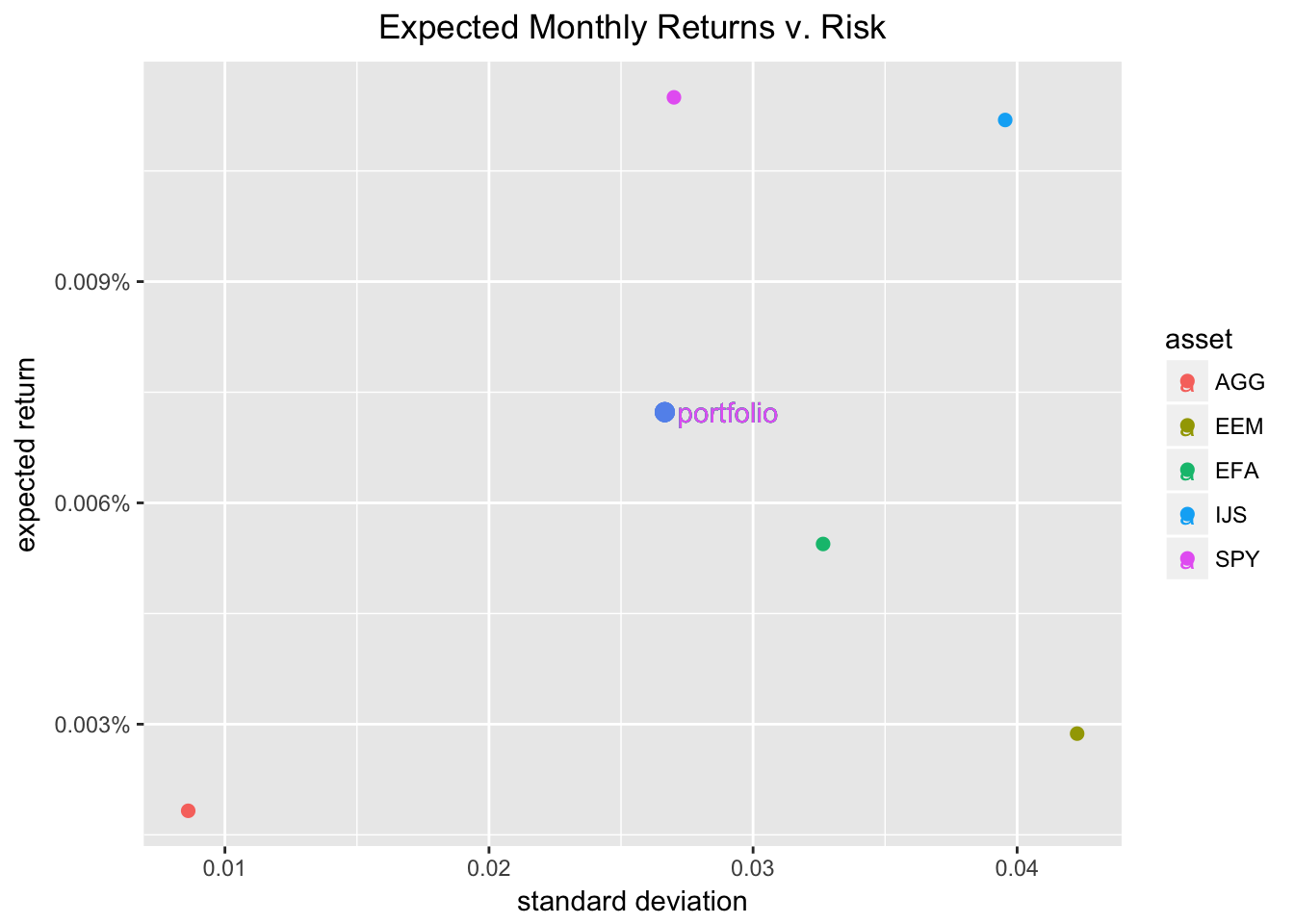Our portfolio return/risk looks all right, though the SP500 has a higher expected return for just a bit more risk. It’s been tough to beat the market the last five years. EEM and EFA have a higher risk and lower expected return (no rational investor wants that!) and IJS has a higher risk and a higher expected return (some rational investors do want that!).

In general, the scatter is providing some return-risk context for our portfolio. It’s not directly part of CAPM, but I like to start here to get in the return-risk mindset.

Next, let’s turn to CAPM more directly and visualize the relationship between our portfolio and the market with a scatter plot of market returns on the x-axis and portfolio returns on the y-axis. First, we will add the market returns to our portfolio tibble by calling mutate(market_returns = market_returns_tidy$returns). Then, we set our x- and y-axis with ggplot(aes(x = market_returns, y = returns)). portfolio_returns_tq_rebalanced_monthly %>% mutate(market_returns = market_returns_tidy$returns) %>%
ggplot(aes(x = market_returns, y = returns)) +
geom_point(color = "cornflowerblue") +
ylab("portfolio returns") +
xlab("market returns") +
ggtitle("Scatterplot of portfolio returns v. market returns")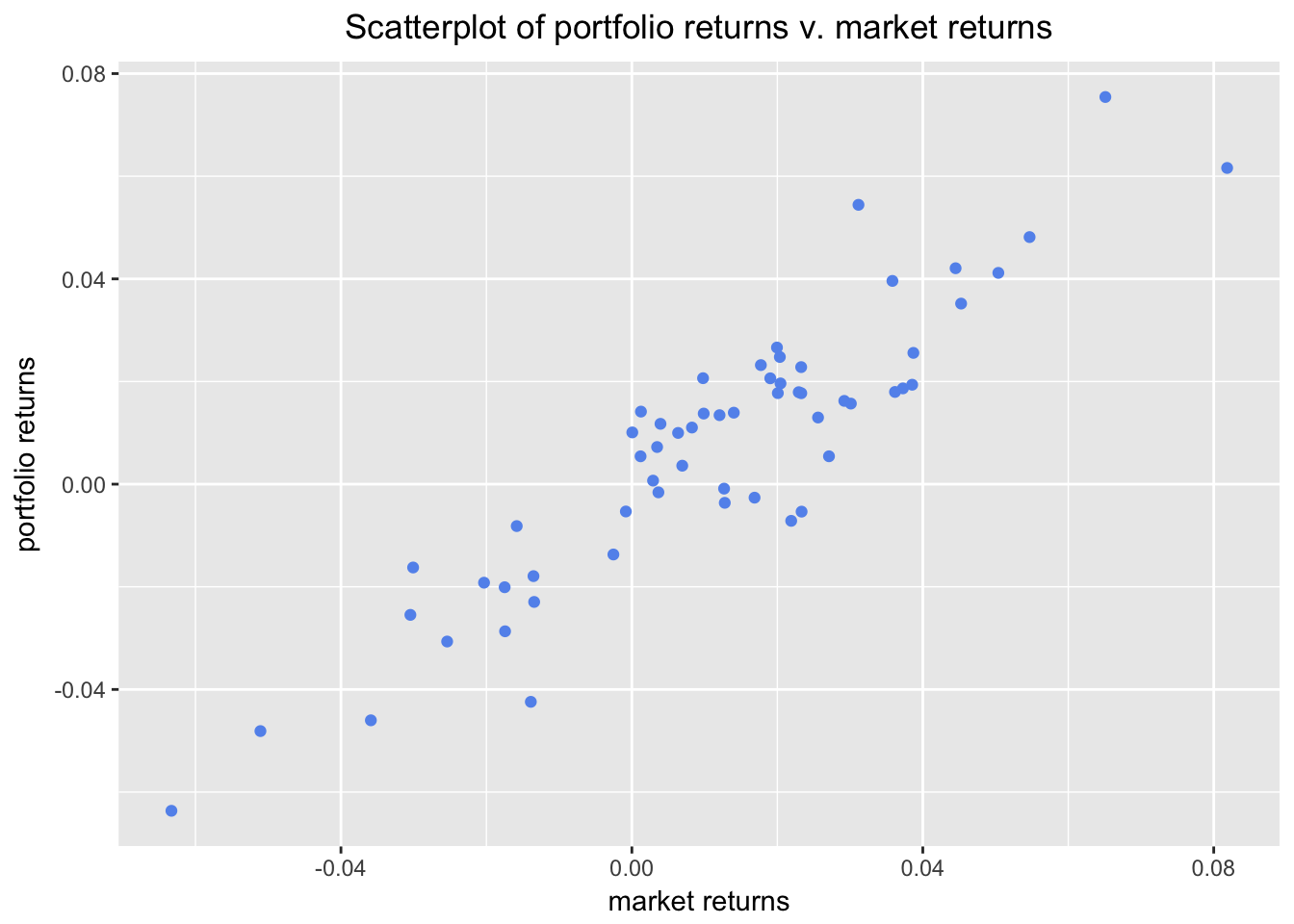This scatter plot is communicating the same strong linear relationship as our numeric beta calculation from the previous post. We can add a simple regression line to it with geom_smooth(method = "lm", se = FALSE, color = "green", size = .5).

portfolio_returns_tq_rebalanced_monthly %>%
mutate(market_returns = market_returns_tidy$returns) %>% ggplot(aes(x = market_returns, y = returns)) + geom_point(color = "cornflowerblue") + geom_smooth(method = "lm", se = FALSE, color = "green", size = .5) + ylab("portfolio returns") + xlab("market returns") + ggtitle("Scatterplot with regression line")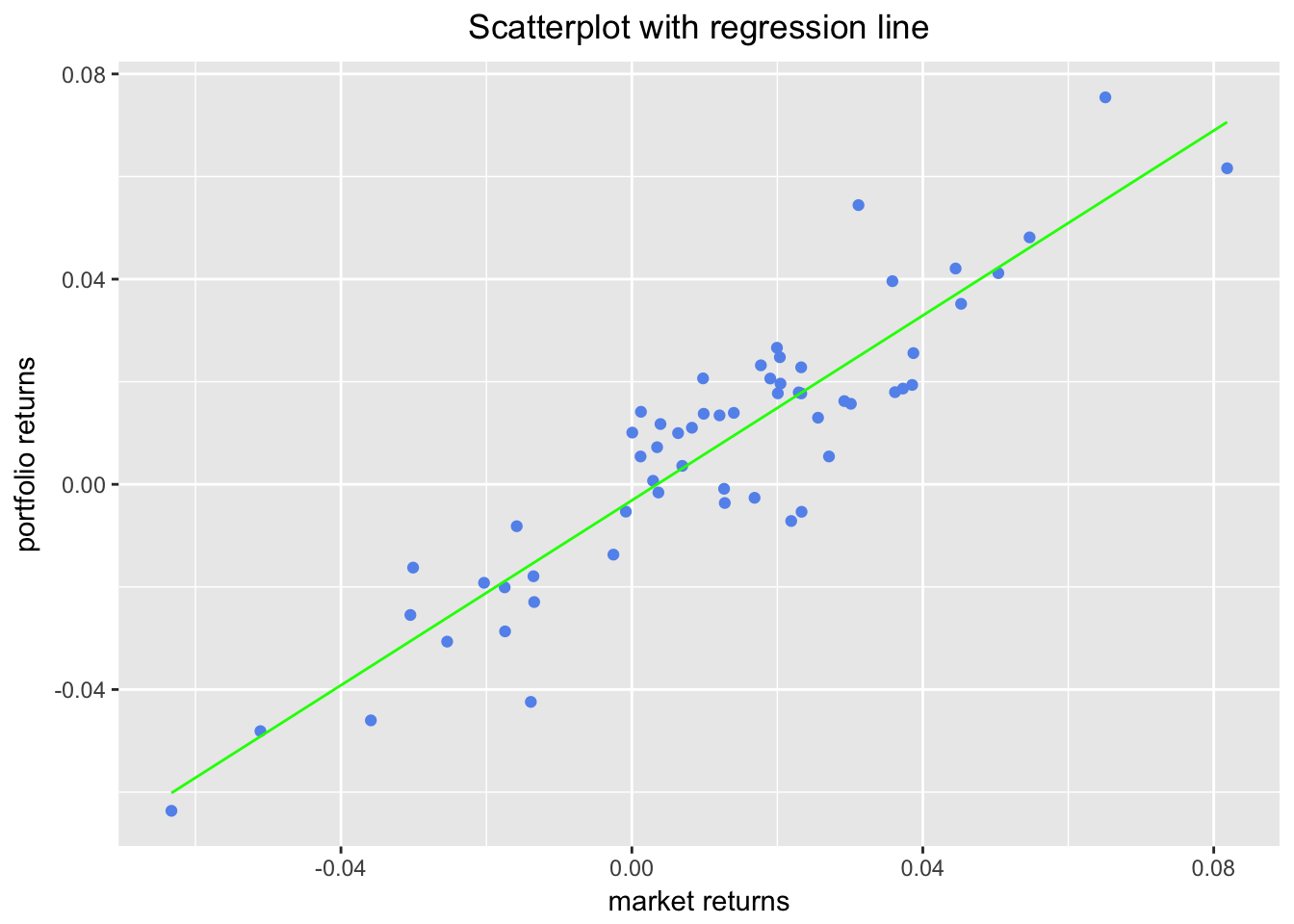The green line is produced by the call to geom_smooth(method = 'lm'). Under the hood, ggplot fits a linear model of the relationship between market returns and portfolio returns. The slope of that green line is the CAPM beta that we calculated earlier. To confirm that we can add a line to the scatter that has a slope equal to our beta calculation and a y-intercept equal to what I labeled as alpha in the beta_dplyr_byhand object. To add the line, we invoke geom_abline(aes(intercept = beta_dplyr_byhand$estimate, slope = beta_dplyr_byhand$estimate), color = "purple"). portfolio_returns_tq_rebalanced_monthly %>% mutate(market_returns = market_returns_tidy$returns) %>%
ggplot(aes(x = market_returns, y = returns)) +
geom_point(color = "cornflowerblue") +
geom_abline(aes(
intercept = beta_dplyr_byhand$estimate, slope = beta_dplyr_byhand$estimate),
color = "purple",
size = .5) +
ylab("portfolio returns") +
xlab("market returns") +
ggtitle("Scatterplot with hand calculated slope")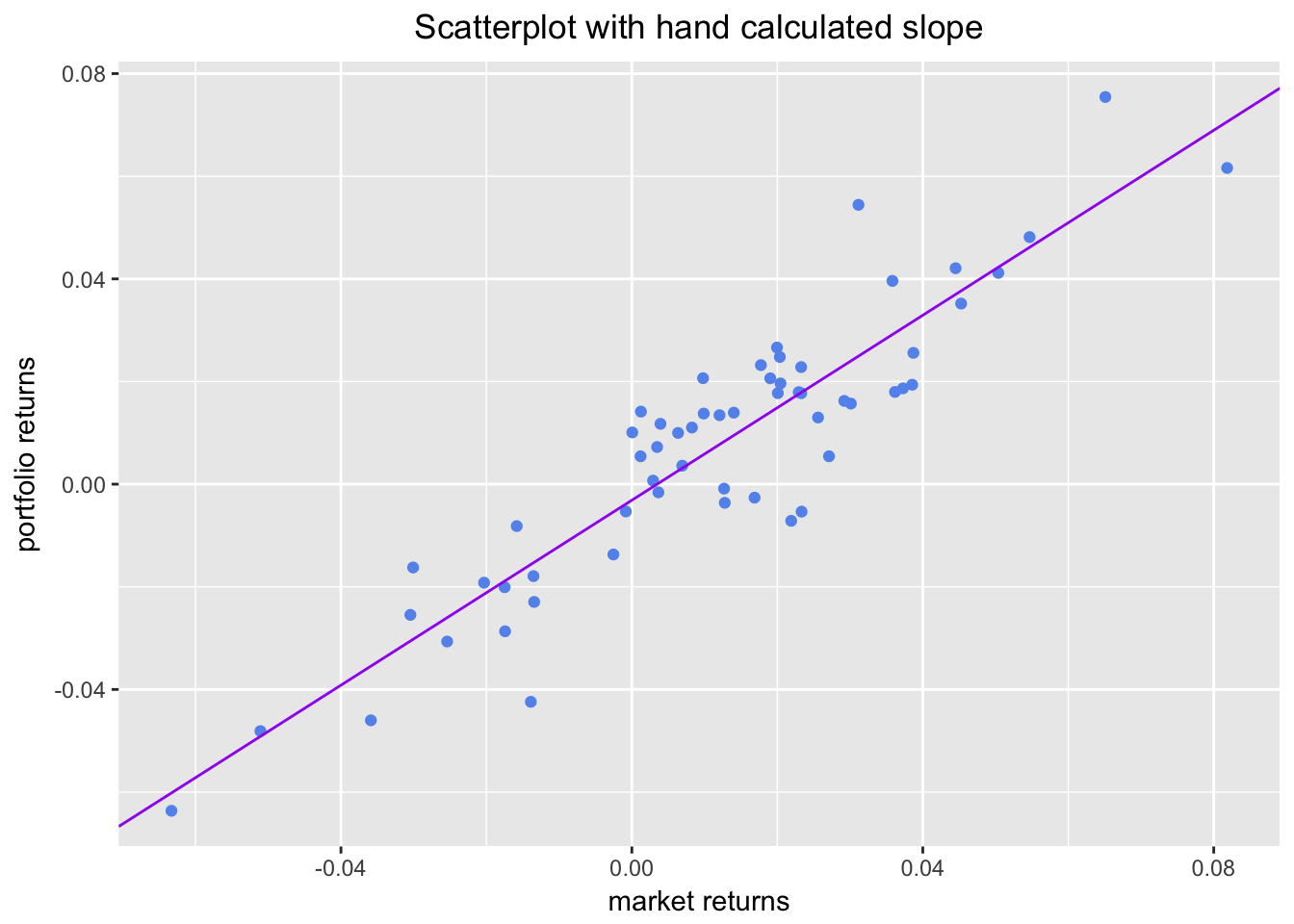We can plot both lines simultaneously to confirm to ourselves that they are the same - they should be right on top of each other but the purple line, our manual abline, extends into infinity so, we should see it start where the green line ends.

portfolio_returns_tq_rebalanced_monthly %>%
mutate(market_returns = market_returns_tidy$returns) %>% ggplot(aes(x = market_returns, y = returns)) + geom_point(color = "cornflowerblue") + geom_abline(aes( intercept = beta_dplyr_byhand$estimate,
slope = beta_dplyr_byhand$estimate), color = "purple", size = .5) + geom_smooth(method = "lm", se = FALSE, color = "green", size = .5) + ylab("portfolio returns") + xlab("market returns") + ggtitle("Compare CAPM beta line to regression line")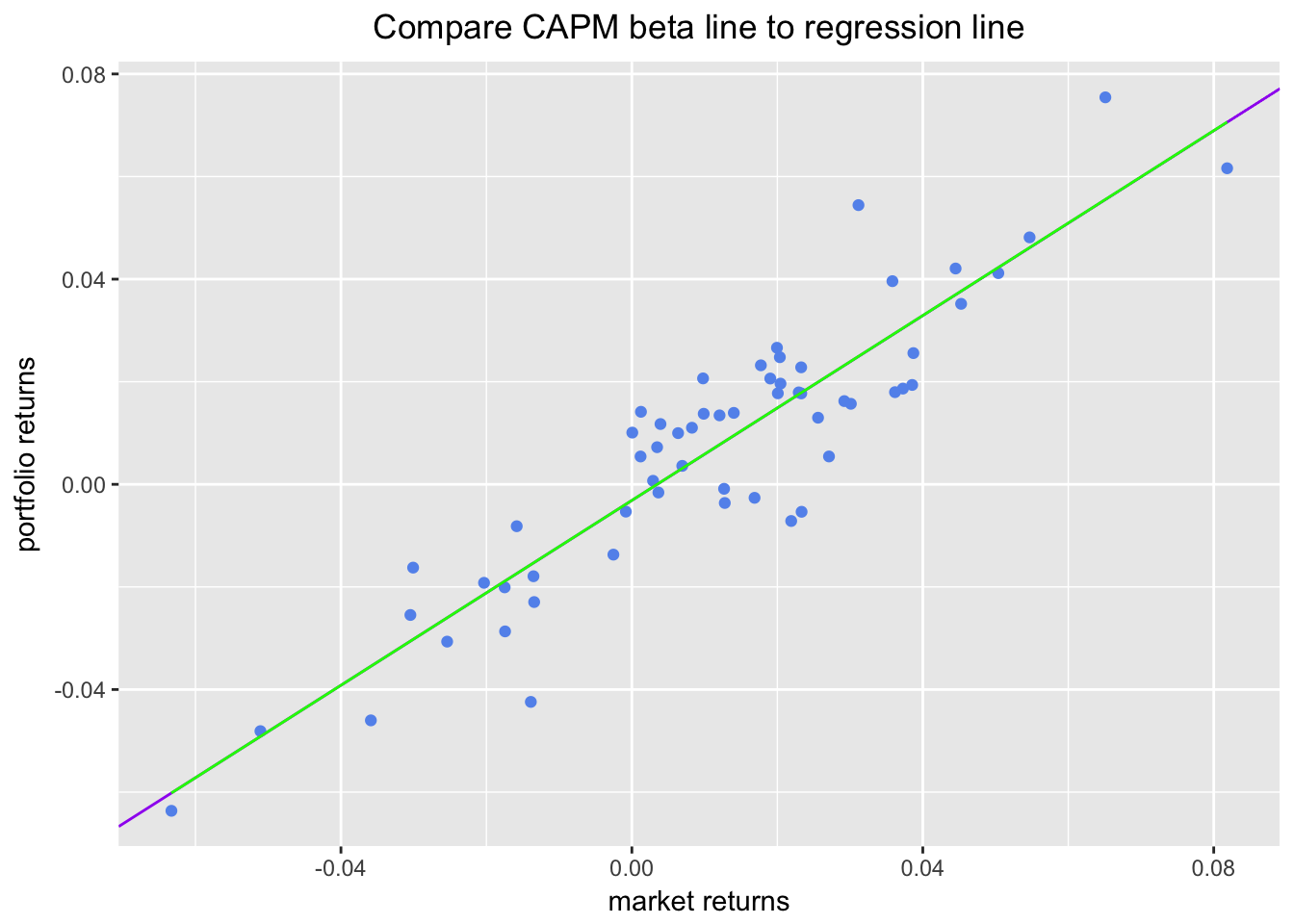All right, that seems to visually confirm (or strongly support) that the fitted line calculated by ggplot and geom_smooth() has a slope equal to the beta we calculated ourselves. Why did we go through this exercise? Well, CAPM beta is a bit “jargony”. Since we need to map that jargon over to the world of linear modeling, it’s a useful practice to consider how jargon reduces to data science concepts. This isn’t a particularly complicated bit of jargon, but it’s good practice to get in the habit of reducing jargon. ### A Bit More on Linear Regression: Augmenting Our Data Before concluding our analysis of CAPM beta, let’s explore the augment() function from broom and how it helps to create a few more interesting visualizations. The code chunk below starts with model results from lm(returns ~ market_returns_tidy$returns...), which is regressing our portfolio returns on the market returns. We store the results in a list-column called called model. Next, we call augment(model) which will add predicted values to the original data set and return a tibble.

Those predicted values will be in the .fitted column. For some reason, the date column gets dropped. It’s nice to have this for visualizations so we will add it back in with mutate(date = portfolio_returns_tq_rebalanced_monthly$date). library(broom) portfolio_model_augmented <- portfolio_returns_tq_rebalanced_monthly %>% do(model = lm(returns ~ market_returns_tidy$returns, data = .))%>%
augment(model) %>%
mutate(date = portfolio_returns_tq_rebalanced_monthly$date) head(portfolio_model_augmented) ## returns market_returns_tidy.returns .fitted .se.fit ## 1 -0.0008696132 0.01267837 0.008294282 0.001431288 ## 2 0.0186624378 0.03726809 0.030451319 0.001984645 ## 3 0.0206248830 0.01903021 0.014017731 0.001485410 ## 4 -0.0053529692 0.02333503 0.017896670 0.001563417 ## 5 -0.0229487590 -0.01343411 -0.015234859 0.001953853 ## 6 0.0411705787 0.05038580 0.042271276 0.002521506 ## .resid .hat .sigma .cooksd .std.resid date ## 1 -0.009163896 0.01698211 0.01101184 0.006116973 -0.8415272 2013-02-28 ## 2 -0.011788881 0.03265148 0.01096452 0.020099701 -1.0913142 2013-03-28 ## 3 0.006607152 0.01829069 0.01104500 0.003434000 0.6071438 2013-04-30 ## 4 -0.023249640 0.02026222 0.01062704 0.047294116 -2.1386022 2013-05-31 ## 5 -0.007713900 0.03164618 0.01103127 0.008323550 -0.7137165 2013-06-28 ## 6 -0.001100697 0.05270569 0.01107986 0.000294938 -0.1029661 2013-07-31 Let’s use ggplot() to see how well the fitted return values match the actual return values. portfolio_model_augmented %>% ggplot(aes(x = date)) + geom_line(aes(y = returns, color = "actual returns")) + geom_line(aes(y = .fitted, color = "fitted returns")) + scale_colour_manual("", values = c("fitted returns" = "green", "actual returns" = "cornflowerblue")) + xlab("date") + ggtitle("Fitted versus actual returns")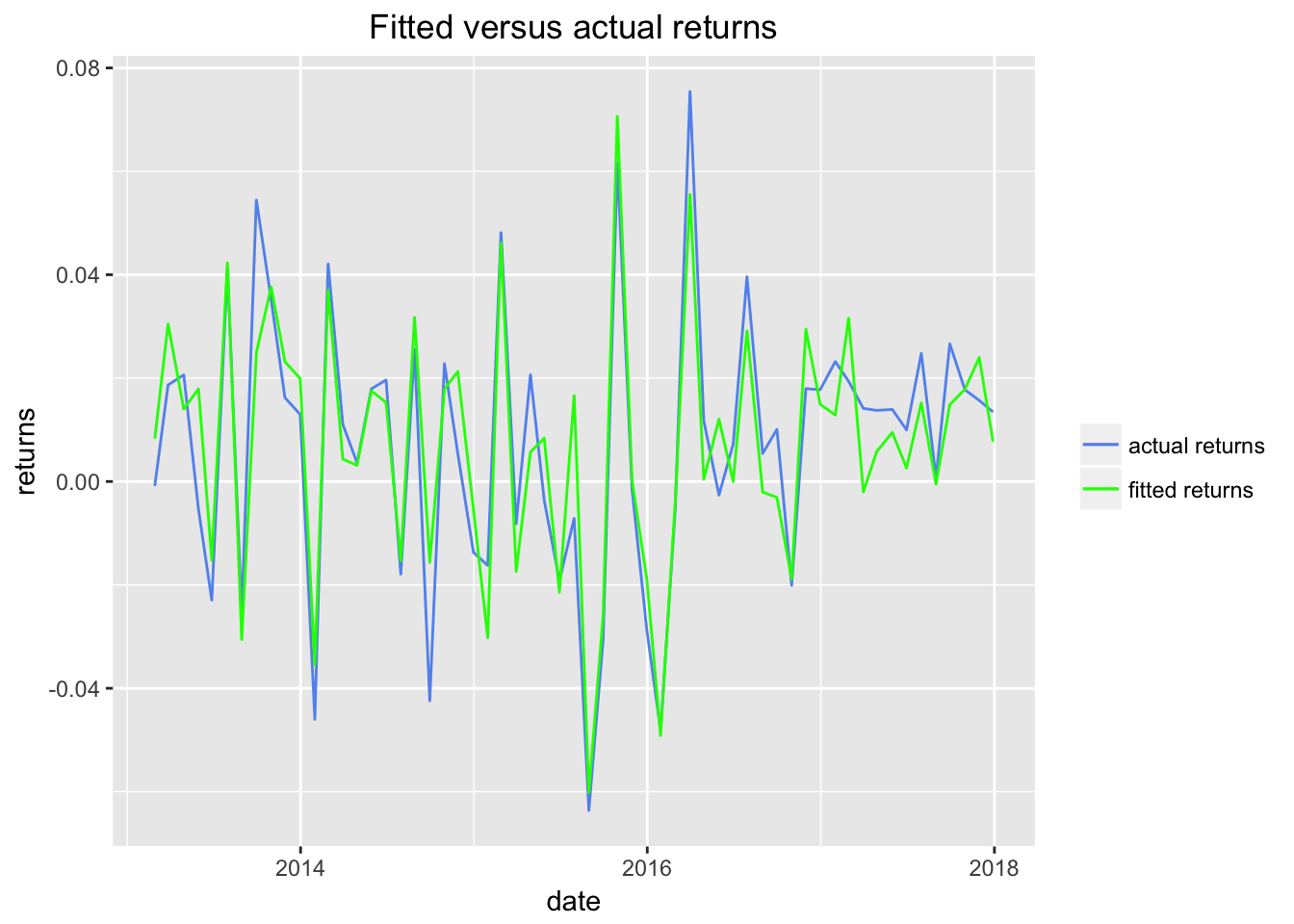Those monthly returns and fitted values seem to track well. Let’s convert both actual returns and fitted returns to the growth of a dollar and run the same comparison. This isn’t a traditional way to visualize actual versus fitted, but it’s still useful. portfolio_model_augmented %>% mutate(actual_growth = cumprod(1 + returns), fitted_growth = cumprod(1 + .fitted)) %>% ggplot(aes(x = date)) + geom_line(aes(y = actual_growth, color = "actual growth")) + geom_line(aes(y = fitted_growth, color = "fitted growth")) + xlab("date") + ylab("actual and fitted growth") + ggtitle("Growth of a dollar: actual v. fitted") + scale_x_date(breaks = pretty_breaks(n = 8)) + scale_y_continuous(labels = dollar) + scale_colour_manual("", values = c("fitted growth" = "green", "actual growth" = "cornflowerblue"))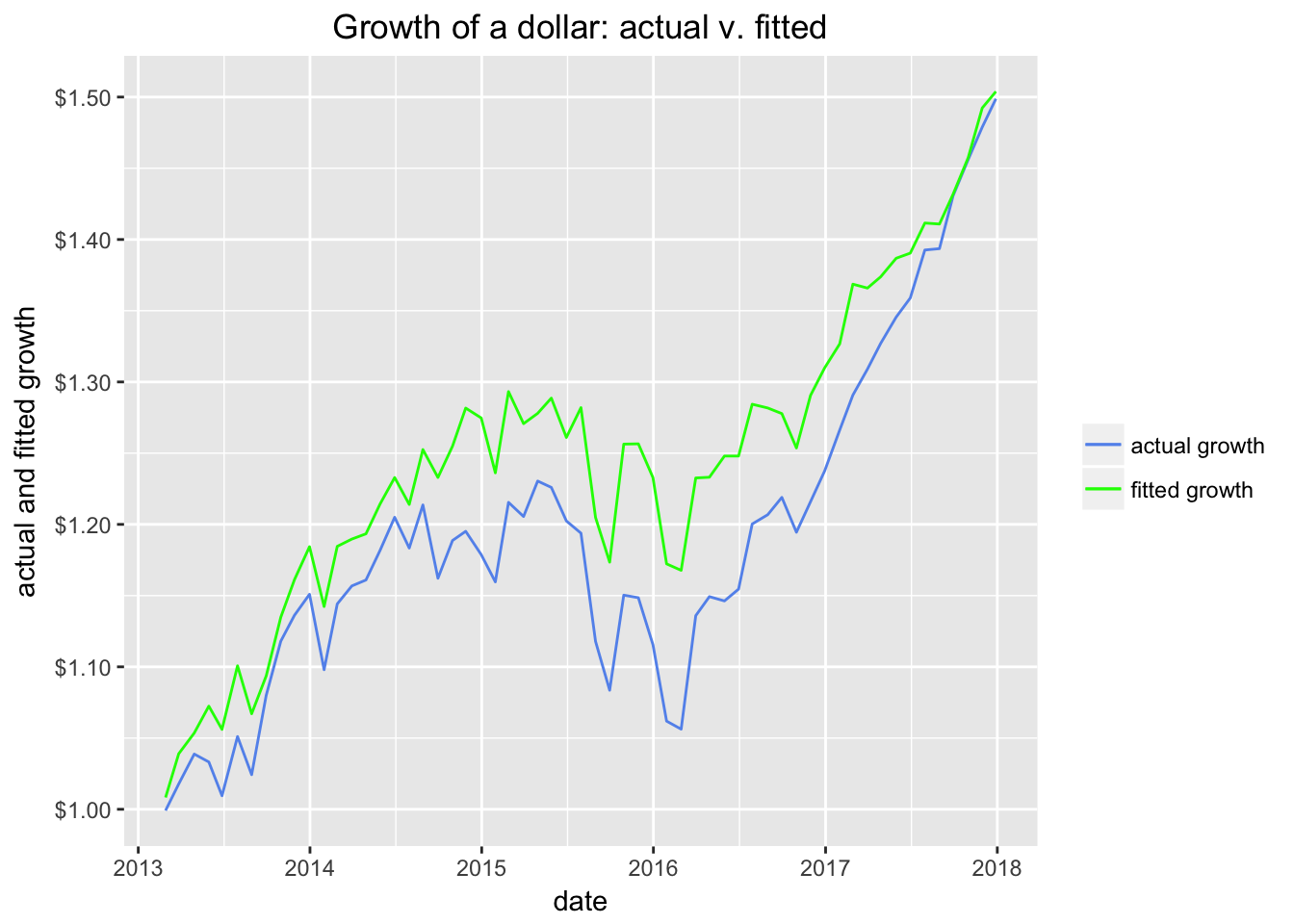Our fitted growth tracks our actual growth well, though the actual growth is lower than predicted for most of the five year history. ### To Highcharter! A nice side benefit of augment() is that it allows us to create an interesting highcharter object that replicates our scatter + regression ggplot from earlier. First, let’s build the base scatter plot of portfolio returns, which are housed in portfolio_model_augmented$returns, against market returns, which are housed in portfolio_model_augmented$market_returns_tidy.returns. library(highcharter) highchart() %>% hc_title(text = "Portfolio v. Market Returns") %>% hc_add_series_scatter(round(portfolio_model_augmented$returns, 4),
round(portfolio_model_augmented$market_returns_tidy.returns, 4)) %>% hc_xAxis(title = list(text = "Market Returns")) %>% hc_yAxis(title = list(text = "Portfolio Returns")) That looks good; but hover over one of the points. If you’re like me, you will desperately wish that the date of the observation were being displayed. Let’s add that date display functionality. First, we need to supply the date observations, so we will add a date variable with hc_add_series_scatter(..., date = portfolio_returns_tq_rebalanced_monthly$date). Then, we want the tool tip to pick up and display that variable. That is done with hc_tooltip(formatter = JS("function(){return ('port return: ' + this.y + ' <br> mkt return: ' + this.x + ' <br> date: ' + this.point.date)}")). We are creating a custom tool tip function to pick up the date. Run the code chunk below and hover over a point.

highchart() %>%
hc_title(text = "Portfolio v. Market Returns") %>%
hc_add_series_scatter(round(portfolio_model_augmented$returns, 4), round(portfolio_model_augmented$market_returns_tidy.returns, 4),
date = portfolio_model_augmented\$date) %>%
hc_xAxis(title = list(text = "Market Returns")) %>%
hc_yAxis(title = list(text = "Portfolio Returns")) %>%
hc_tooltip(formatter = JS("function(){
return ('port return: ' + this.y + ' <br> mkt return: ' + this.x +
' <br> date: ' + this.point.date)}"))

I was curious about the most negative reading in the bottom left, and this new tool tip makes it easy to see that it occurred in August of 2015.

Finally, let’s add the regression line.

To do that, we need to supply x and y coordinates to highcharter and specify that we want to add a line instead of more scatter points. We have the x and y coordinates for our fitted regression line because we added them with the augment() function. The x’s are the market returns and the y’s are the fitted values. We add this element to our code flow with hc_add_series(portfolio_model_augmented, type = "line", hcaes(x = market_returns_tidy.returns, y = .fitted), name = "CAPM Beta = Regression Slope")

highchart() %>%
hc_title(text = "Scatter with Regression Line") %>%
type = "scatter",
hcaes(x = round(market_returns_tidy.returns, 4),
y = round(returns, 4),
date = date),
name = "Returns") %>%
type = "line",
hcaes(x = market_returns_tidy.returns, y = .fitted),
name = "CAPM Beta = Regression Slope") %>%
hc_xAxis(title = list(text = "Market Returns")) %>%
hc_yAxis(title = list(text = "Portfolio Returns")) %>%
hc_tooltip(formatter = JS("function(){
return ('port return: ' + this.y + ' <br> mkt return: ' + this.x +
' <br> date: ' + this.point.date)}"))

That’s all for today and thanks for reading.